Written by Paul Bourke
April 2001

Chladni plate interference surfaces are defined as positions where N harmonics cancel. Instead of restricting this to a line or plane as in classical Chladni's plate experiments, a rich set of surfaces result from having 3 orthogonal harmonics as follows:

cos(c1 x) + cos(c2 y) + cos(c3 z) = 0

Where 0 < x < pi, 0 < y < pi, and 0 < z < pi. The surface is periodic outside this domain. The table below gives a selected set of the c1, c2, c3 space from 0.5 to 3.0. Note from symmetry cases where the "c" are the same but in a different order need not be shown since they are rotations or reflections of each other. The gaps in the table below would contain images already shown in other cells. The first row increases the "c" parameters together. The remaining rows consist of c3 increasing horizontally and c2 increasing vertically. Click on the images for higher resolution versions.

 c1 = 0.5, c2 = 0.5, c3 = 0.5 c1 = 1.0, c2 = 1.0, c3 = 1.0 c1 = 2.0, c2 = 2.0, c3 = 2.0 c1 = 3.0, c2 = 3.0, c3 = 3.0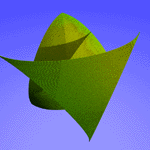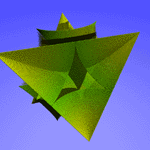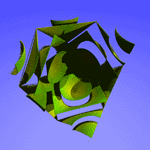c1 = 1.0, c2 = 1.0, c3 = 1.0 c1 = 1.0, c2 = 1.5, c3 = 1.0 c1 = 1.0, c2 = 2.0, c3 = 1.0 c1 = 1.0, c2 = 2.5, c3 = 1.0c1 = 1.0, c2 = 1.0, c3 = 1.25 c1 = 1.0, c2 = 1.5, c3 = 1.25 c1 = 1.0, c2 = 2.0, c3 = 1.25 c1 = 1.0, c2 = 2.5, c3 = 1.25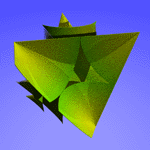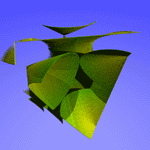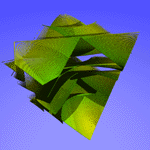c1 = 1.0, c2 = 1.0, c3 = 1.5 c1 = 1.0, c2 = 1.5, c3 = 1.5 c1 = 1.0, c2 = 2.0, c3 = 1.5 c1 = 1.0, c2 = 2.5, c3 = 1.5 Seec1 = 1.0, c2 = 1.5, c3 = 1.0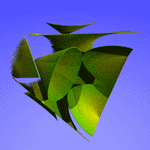c1 = 1.0, c2 = 1.0, c3 = 1.75 c1 = 1.0, c2 = 1.5, c3 = 1.75 c1 = 1.0, c2 = 2.0, c3 = 1.75 c1 = 1.0, c2 = 2.5, c3 = 1.75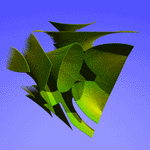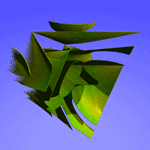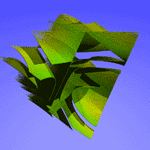c1 = 1.0, c2 = 1.0, c3 = 2.0 c1 = 1.0, c2 = 1.5, c3 = 2.0 c1 = 1.0, c2 = 2.0, c3 = 2.0 c1 = 1.0, c2 = 2.5, c3 = 2.0 Seec1 = 1.0, c2 = 2.0, c3 = 1.0 Seec1 = 1.0, c2 = 2.5, c3 = 1.0c1 = 1.0, c2 = 1.0, c3 = 2.25 c1 = 1.0, c2 = 1.5, c3 = 2.25 c1 = 1.0, c2 = 2.0, c3 = 2.25 c1 = 1.0, c2 = 2.5, c3 = 2.25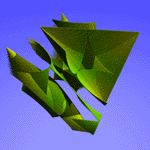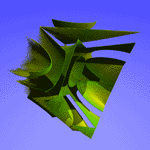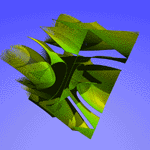Written by Paul Bourke
March 2003

The basic experiment that is given the name "Chladni" consists of a plate or drum of some shape, possibly constrained at the edges or at a point in the center, and forced to vibrate historically with a violin bow or more recently with a speaker. A fine sand or powder is sprinkled on the surface and it is allowed to settle. It will do so at those parts of the surface that are not vibrating, namely at the nodes of vibration.

The equation for the zeros of the standing wave on a square Chladni plate (side length L) constrained at the center is given by the following.

cos(n pi x / L) cos(m pi y / L) - cos(m pi x / L) cos(n pi y / L) = 0

where n and m are integers. The Chladni patterns for n,m between 1 and 5 are shown below, click on the image for a larger version or click on the "continuous" link for the standing wave amplitude maps. Note that the solution is uninteresting for n = m and the lower half of the table is the same as the upper half, namely (n1,m2) = (n2,m1).

Without the constraint in the center the modes are somewhat less interesting, the results are sown below for m=1 and n=1 to 4. The solution is given by:

sin(n pi x / Lx) sin(n pi y / Ly) = 0

Circular plate

For a circular plate with radius R the solution is given in terms of polar coordinates (r,theta) by

Jn(K r) (C1 cos(n theta) + C2 sin(n theta))

Where Jn is the n'th order Bessel function. If the plate is fixed around the rim (eg: a drum) then K = Znm / R, Znm is the m'th zero of the n'th order Bessel function. The term "Znm r / R" means the Bessel function term goes to zero at the rim as required by the constraint of the rim being fixed.

Some examples of the node of a circular plate are given below.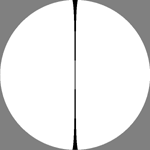(1,1) Continuous(1,3) Continuous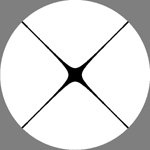(2,1) Continuous(2,4) Continuous

History

Ernest Florens Friedrich Chladni (1756 - 1827) performed many experiments to study the nodes of vibration of circular and square plates, generally fixed in the center and driven with a violin bow. The modes of vibration were identified by scattering salt or sand on the plate, these small particles end up in the places of zero vibration. Ernst Chladni first demonstrated this at the French Academy of Science in 1808, it caused such interest that the Emperor offered a kilogram of gold to the first person who could explain the patterns. The following is a drawing from Chladni's original publication.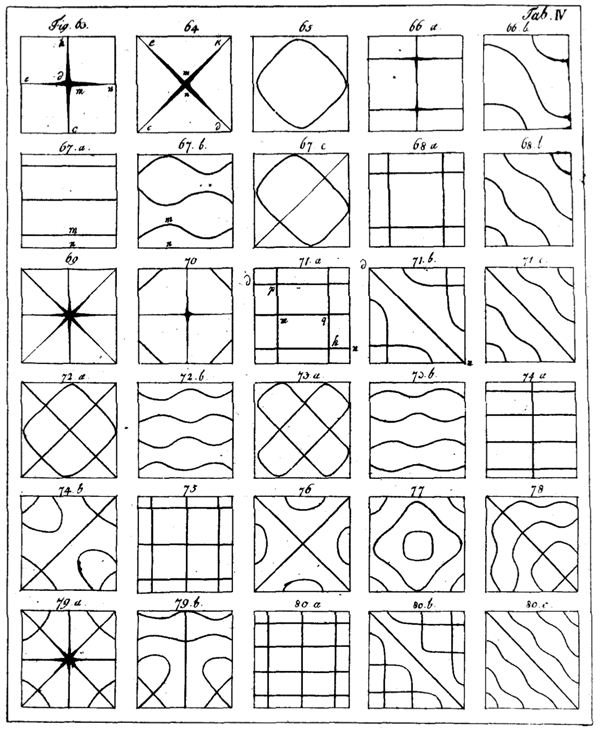Contribution by Nikola Nikolov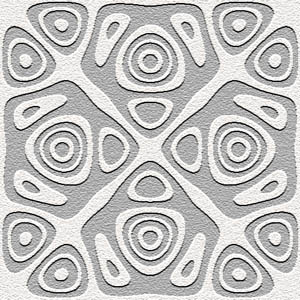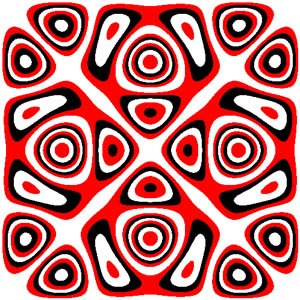References

Rossing, Thomas D., Chladni's Law for Vibrating Plates. American Journal of Physics.Vol 50. no 3. March, 1982

William C. Elmore and Mark A. Heald. Physics of Waves. New York: Dover Publications.

Hutchins, C.M., The acoustics of violin plates. Scientific American, Oct.1981, 170-176.

Fletcher, N.H. & Rossing,T.D., The Physics of Musical Instruments., Springer-Verlag, New York, 1991.